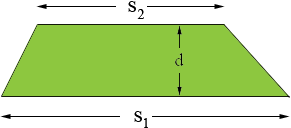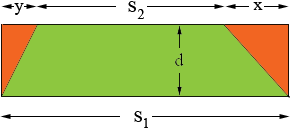SEARCH HOMEMath Central Quandaries & QueriesQuestion from Ashley, a student: what is the formula for area of a trapezoidAshley,

I remember the area of a trapezoid as

the average of the lengths of the parallel sides times the distance between the parallel sides.

I can show you why this is true for one type of trapezoid and leave it to you to check for other trapezoids.

Suppose I have a trapezoid where both base angles are acute. Let s1 and s2 be the lengths of the parallel sides with s1s2and d be the distance between the parallel sides.Construct a rectangle with base s1 and height d. The area of the trapezoid is the area of this rectangle minus the areas of the two triangles shaded orange in the diagram.That is

trapezoid area = s1 × d - 1/2 d × x - 1/2 d × y
= d × (s1 - 1/2(x + y))

But s1 - s2 = x + y so

trapezoid area = d × (s1 - 1/2(s1 - s2))
= 1/2 (s1 + s2) × d.

PennyMath Central is supported by the University of Regina and The Pacific Institute for the Mathematical Sciences.